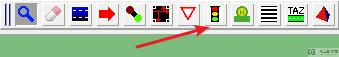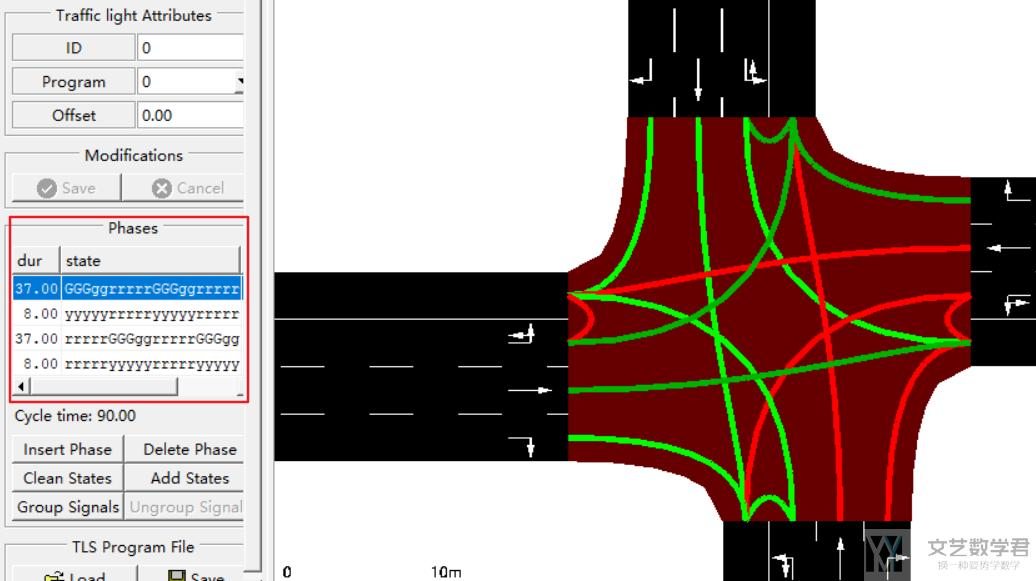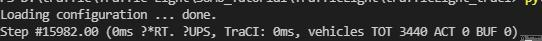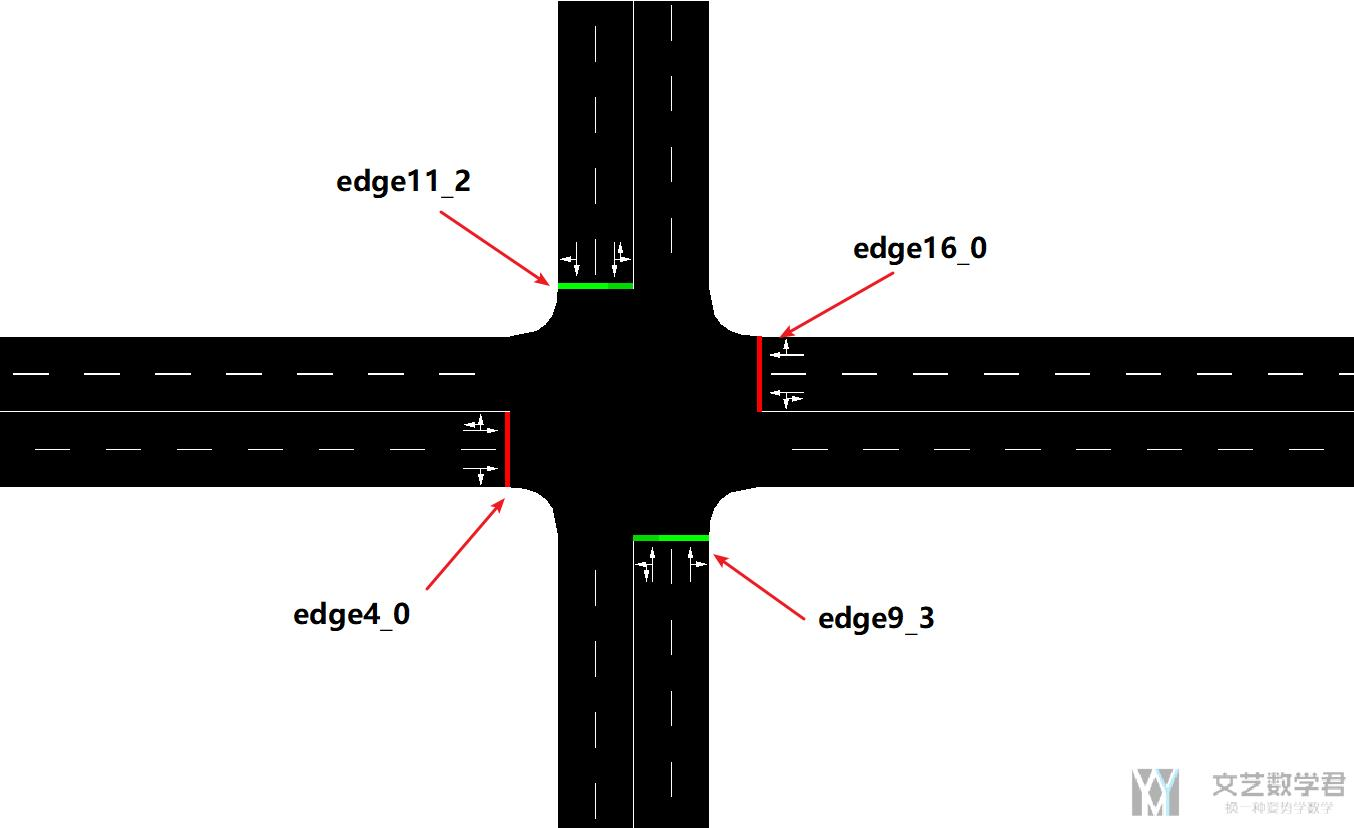# 使用 SUMO 进行仿真(4)-信号灯（Traffic Light）2021年9月26日07:09:05

5 6390字阅读21分18秒

## Traffic Light 的介绍

1. <node id="0" x="0.0" y="0.0" type="traffic_light"/>

2.     <tlLogic id="0" type="static" programID="0" offset="0">
3.         <phase duration="37" state="GGGggrrrrrGGGggrrrrr"/> <!--绿灯-->
4.         <phase duration="8"  state="yyyyyrrrrryyyyyrrrrr"/> <!--黄灯-->
5.         <phase duration="37" state="rrrrrGGGggrrrrrGGGgg"/> <!--红灯-->
6.         <phase duration="8"  state="rrrrryyyyyrrrrryyyyy"/> <!--黄灯-->
7.     </tlLogic>

• id 为节点 id，即这个信号灯会设置在哪个节点上；
• programID 是该节点一套信号配时方案的 ID 名。这代表在一个节点可以设置多套信号配时方案。这为后续的实时控制打下了基础。
• offset 则为信号灯启动的时间。
• type 代表了信号灯的类型(static，actuated)，其中 static 代表固定相位的信号灯，actuated 则可根据车流状况适当延长与缩短相位，类似感应控制。

`netconvert --node-files=hello.nod.xml --edge-files=hello.edg.xml --tllogic-files=hello.add.xml --output-file=hello.net.xml`## 使用 TraCI 控制信号灯

1. import os
2. import sys
3. # 确保 traci 在系统环境变量中
4. if 'SUMO_HOME' in os.environ:
5.     tools = os.path.join(os.environ['SUMO_HOME'], 'tools')
6.     sys.path.append(tools)
7.     print('SUMO_HOME is In Environment!')
8. else:
9.     sys.exit("please declare environment variable 'SUMO_HOME'")

### 使用 TraCI 开启并运行仿真

1. import sys
2. import traci
3. class Sim(object):
4.     def __init__(self, sumo_config, GUI=False):
5.         self.sumo_config = sumo_config # sumo config 文件
6.         self.launch_env_flag = False
7.         self.GUI = GUI
8.     def launchEnv(self):
9.         """开始模拟(通过traci来获得其中数据)
10.         """
11.         if self.GUI:
12.             sumo_gui = 'sumo-gui'
13.         else:
14.             sumo_gui = 'sumo'
15.         traci.start([
16.             sumo_gui,
17.             "-c", self.sumo_config,
18.             "--no-warnings",
19.             "--seed", "2"])
20.         self.launch_env_flag = True
21.     def close(self):
22.         """关闭实验环境
23.         """
24.         traci.close()
25.         self.launch_env_flag = False
26.         sys.stdout.flush()
27.     def reset(self):
28.         """关闭当前环境, 并开启一个新的环境
29.         """
30.         self.close()
31.         self.launchEnv()
32.     def step(self):
33.         steps = 0
34.         assert self.launch_env_flag
35.         while traci.simulation.getMinExpectedNumber() > 0: # 当路网里面还有车
36.             traci.simulationStep()
37.             steps = steps + 1
38.     def runSim(self):
39.         """开始模拟
40.         """
41.         self.launchEnv()  # 初始化环境
42.         self.step()  # 进行模拟
43.         self.close()  # 关闭环境
44. if __name__ == '__main__':
45.     sumo_sim = Sim(sumo_config='./env/hello.sumocfg')
46.     sumo_sim.runSim()### 使用 TraCI 获得信号灯的信息

1. print(traci.trafficlight.getNextSwitch('haxl_htxdj')) # 到下一次切换信号灯的时间
2. print(traci.trafficlight.getPhaseDuration('haxl_htxdj')) # 目前这个phase的持续时间

1. traci.trafficlight.getCompleteRedYellowGreenDefinition

1. Logic(programID='0', type=0, currentPhaseIndex=0, phases=(
2.     Phase(duration=35.0, state='GGGrrrrrrGGGGrrrrr', minDur=35.0, maxDur=35.0, next=()),
3.     Phase(duration=5.0, state='yyyrrrrrryyyyrrrrr', minDur=5.0, maxDur=5.0, next=()),
4.     Phase(duration=6.0, state='rrrGrrrrrrrrrGrrrr', minDur=6.0, maxDur=6.0, next=()),
5.     Phase(duration=5.0, state='rrryrrrrrrrrryrrrr', minDur=5.0, maxDur=5.0, next=()),
6.     Phase(duration=5.0, state='rrrrrrrrrrrrrrrrrr', minDur=5.0, maxDur=5.0, next=()),
7.     Phase(duration=34.0, state='rrrrGGGGgrrrrrGGGg', minDur=34.0, maxDur=34.0, next=()),
8.     Phase(duration=5.0, state='rrrryyyyyrrrrryyyy', minDur=5.0, maxDur=5.0, next=()),
9.     Phase(duration=5.0, state='rrrrrrrrrrrrrrrrrr', minDur=5.0, maxDur=5.0, next=())),
10.     subParameter={}),

### 使用 Traci 设置信号灯信息

• 其中 tlsID 表示信号灯的 ID。
• 其中 tls 是一个 Logic 类型的数据，实际使用的时候，我们可以通过 `getCompleteRedYellowGreenDefinition` 获得数据，接着对 Logic 类型的数据进行修改即可。

1. import traci
2. from sim import Sim
3. sumo_sim = Sim(sumo_config='./env/hello.sumocfg')
4. sumo_sim.launchEnv() # 开启仿真
5. # 获取信号灯信息
6. logic_list = traci.trafficlight.getCompleteRedYellowGreenDefinition('0') # 只有一个 program
7. print(logic_list)
8. # 修改信号灯信息, 将绿灯时长都加 10 秒
9. for phase in logic_list.phases:
10.     if 'G' in phase.state:
11.         phase.duration += 10
12. traci.trafficlight.setCompleteRedYellowGreenDefinition(tlsID='0', tls=logic_list)
13. # 重新打印修改后的红绿灯信息
14. logic_list = traci.trafficlight.getCompleteRedYellowGreenDefinition('0')
15. print(logic_list)

### TraCI 控制信号灯变化例子-根据车流数量进行调整• traci.edge.getLastStepVehicleNumber 获得指定 edge 的车辆的数量；
• traci.trafficlight.setRedYellowGreenState 修改某个 node 的当前的信号灯状态；

1. import traci
2. import time
3. # traci.start其实就是将sumo的命令行指令以列表形式读取, 运行sumo-gui程序, 其指令方式与cmd命令行相同
4. traci.start(["C:/Program Files (x86)/Eclipse/Sumo/bin/sumo-gui.exe","-c","D:/cross.sumocfg"])
5. step=0
6. while step<3600:
7.     traci.simulationStep() # 每次触发一次
8.     # 通过time.sleep命令降低仿真的速度
9.     time.sleep(0.1)
10.     step=step+1
11.     # 比较路段车辆数多少,改变信号灯相位状态
12.     if traci.edge.getLastStepVehicleNumber('edge4_0')+traci.edge.getLastStepVehicleNumber('edge16_0')>traci.edge.getLastStepVehicleNumber('edge11_2')+traci.edge.getLastStepVehicleNumber('edge9_3'):
13.         traci.trafficlight.setRedYellowGreenState('node10','GGGggrrrrrGGGggrrrrr') # 设置node的红绿灯状态
14.         print('绿灯状态')
15.     else:
16.         traci.trafficlight.setRedYellowGreenState('node10','rrrrrGGGggrrrrrGGGgg')
17. traci.close() # 关闭仿真

• 微信公众号
• 关注微信公众号
•• QQ群
• 我们的QQ群号
•• 本文由 发表于 2021年9月26日07:09:05
• 转载请务必保留本文链接：https://mathpretty.com/14088.html